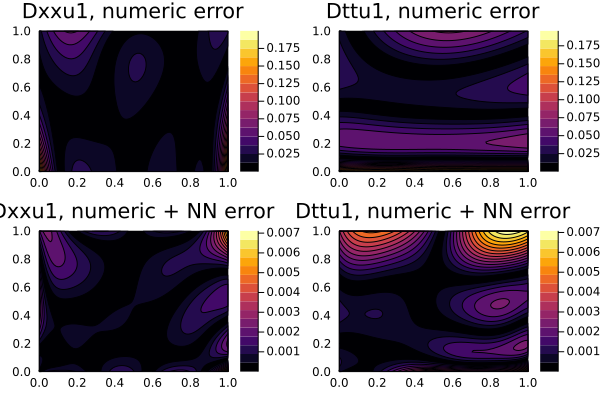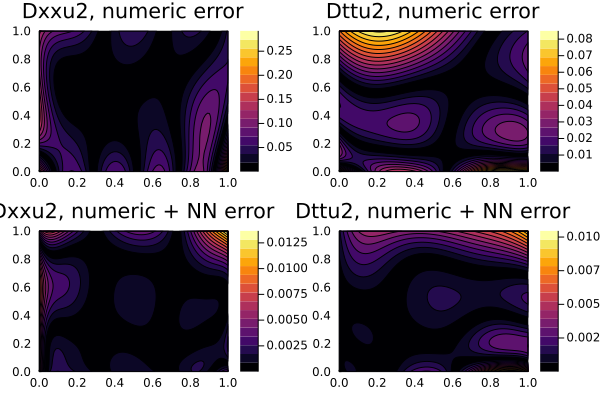# The Derivative neural network approximation

The accuracy and stability of numerical derivative decreases with each successive order. The accuracy of the entire solution is determined by the worst accuracy of one of the variables, in our case - the highest degree of the derivative. Meanwhile the computational cost of automatic differentation for higher orders grows the power in O(n^d), making even numerical differentiation much more efficient! Given these two bad choices, there exists an alternative which can improve training speed and accuracy: using a system to represent the derivatives directly.

## Demonstration

Take the PDE system:

\begin{align*} ∂_t u_1(t, x) & = ∂_x^2 u_1(t, x) + u_3(t, x) \, \sin(\pi x) \, ,\\ ∂_t u_2(t, x) & = ∂_x^2 u_2(t, x) + u_3(t, x) \, \cos(\pi x) \, ,\\ 0 & = u_1(t, x) \sin(\pi x) + u_2(t, x) \cos(\pi x) - e^{-t} \, , \end{align*}

with the initial conditions:

\begin{align*} u_1(0, x) & = \sin(\pi x) \, ,\\ ∂_t u_1(0, x) & = - \sin(\pi x) \, ,\\ u_2(0, x) & = \cos(\pi x) \, ,\\ ∂_t u_2(0, x) & = - \cos(\pi x) \, , \end{align*}

and the boundary conditions:

\begin{align*} u_1(t, 0) & = u_1(t, 1) = 0 \, ,\\ u_2(t, 0) & = - u_2(t, 1) = e^{-t} \, , \end{align*}

This is the same system as the system of equations example

The derivative neural network approximation is such an approach that using lower-order numeric derivatives and estimates higher-order derivatives with a neural network so that allows an increase in the marginal precision for all optimization. Since u3 is only in the first and second equations, that its accuracy during training is determined by the accuracy of the second numerical derivative u3(t,x) ~ (Dtt(u1(t,x)) -Dxx(u1(t,x))) / sin(pi*x).

We approximate the derivative of the neural network with another neural network Dt(u1(t,x)) ~ Dtu1(t,x) and train it along with other equations, and thus we avoid using the second numeric derivative Dt(Dtu1(t,x)).

using NeuralPDE, Lux, ModelingToolkit
using Optimization, OptimizationOptimisers, OptimizationOptimJL
using Plots
import ModelingToolkit: Interval, infimum, supremum

@parameters t, x
Dt = Differential(t)
Dx = Differential(x)
@variables u1(..), u2(..), u3(..)
@variables Dxu1(..) Dtu1(..) Dxu2(..) Dtu2(..)

eqs_ = [Dt(Dtu1(t,x)) ~ Dx(Dxu1(t,x)) + u3(t,x)*sin(pi*x),
Dt(Dtu2(t,x)) ~ Dx(Dxu2(t,x)) + u3(t,x)*cos(pi*x),
exp(-t) ~ u1(t,x)*sin(pi*x) + u2(t,x)*cos(pi*x)]

bcs_ = [u1(0.,x) ~ sin(pi*x),
u2(0.,x) ~ cos(pi*x),
Dt(u1(0,x)) ~ -sin(pi*x),
Dt(u2(0,x)) ~ -cos(pi*x),
#Dtu1(0,x) ~ -sin(pi*x),
# Dtu2(0,x) ~ -cos(pi*x),
u1(t,0.) ~ 0.,
u2(t,0.) ~ exp(-t),
u1(t,1.) ~ 0.,
u2(t,1.) ~ -exp(-t)]

der_ = [Dt(u1(t,x)) ~ Dtu1(t,x),
Dt(u2(t,x)) ~ Dtu2(t,x),
Dx(u1(t,x)) ~ Dxu1(t,x),
Dx(u2(t,x)) ~ Dxu2(t,x)]

bcs__ = [bcs_;der_]

# Space and time domains
domains = [t ∈ Interval(0.0, 1.0),
x ∈ Interval(0.0, 1.0)]

input_ = length(domains)
n = 15
chain = [Lux.Chain(Dense(input_,n,Lux.σ),Dense(n,n,Lux.σ),Dense(n,1)) for _ in 1:7]

grid_strategy = NeuralPDE.GridTraining(0.07)
discretization = NeuralPDE.PhysicsInformedNN(chain,
grid_strategy)

vars = [u1(t,x),u2(t,x),u3(t,x),Dxu1(t,x),Dtu1(t,x),Dxu2(t,x),Dtu2(t,x)]
@named pdesystem = PDESystem(eqs_,bcs__,domains,[t,x],vars)
prob = NeuralPDE.discretize(pdesystem,discretization)
sym_prob = NeuralPDE.symbolic_discretize(pdesystem,discretization)

pde_inner_loss_functions = sym_prob.loss_functions.pde_loss_functions
bcs_inner_loss_functions = sym_prob.loss_functions.bc_loss_functions[1:7]
aprox_derivative_loss_functions = sym_prob.loss_functions.bc_loss_functions[9:end]

callback = function (p,l)
println("loss: ", l )
println("pde_losses: ", map(l_ -> l_(p), pde_inner_loss_functions))
println("bcs_losses: ", map(l_ -> l_(p), bcs_inner_loss_functions))
println("der_losses: ", map(l_ -> l_(p), aprox_derivative_loss_functions))
return false
end

res = Optimization.solve(prob, Adam(0.01); callback = callback, maxiters=2000)
prob = remake(prob,u0=res.u)
res = Optimization.solve(prob,BFGS(); callback = callback, maxiters=10000)

phi = discretization.phi

And some analysis:

using Plots

ts,xs = [infimum(d.domain):0.01:supremum(d.domain) for d in domains]
minimizers_ = [res.u.depvar[sym_prob.depvars[i]] for i in 1:length(chain)]

u1_real(t,x) = exp(-t)*sin(pi*x)
u2_real(t,x) = exp(-t)*cos(pi*x)
u3_real(t,x) = (1+pi^2)*exp(-t)
Dxu1_real(t,x) = pi*exp(-t)*cos(pi*x)
Dtu1_real(t,x) = -exp(-t)*sin(pi*x)
Dxu2_real(t,x) = -pi*exp(-t)*sin(pi*x)
Dtu2_real(t,x) = -exp(-t)*cos(pi*x)
analytic_sol_func_all(t,x) = [u1_real(t,x), u2_real(t,x), u3_real(t,x),
Dxu1_real(t,x),Dtu1_real(t,x),Dxu2_real(t,x),Dtu2_real(t,x)]

u_real  = [[analytic_sol_func_all(t,x)[i] for t in ts for x in xs] for i in 1:7]
u_predict  = [[phi[i]([t,x],minimizers_[i]) for t in ts  for x in xs] for i in 1:7]
diff_u = [abs.(u_real[i] .- u_predict[i] ) for i in 1:7]

titles = ["u1","u2","u3","Dtu1","Dtu2","Dxu1","Dxu2"]
for i in 1:7
p1 = plot(ts, xs, u_real[i], linetype=:contourf,title = "$(titles[i]), analytic"); p2 = plot(ts, xs, u_predict[i], linetype=:contourf,title = "predict"); p3 = plot(ts, xs, diff_u[i],linetype=:contourf,title = "error"); plot(p1,p2,p3) savefig("3sol_ub$i")
end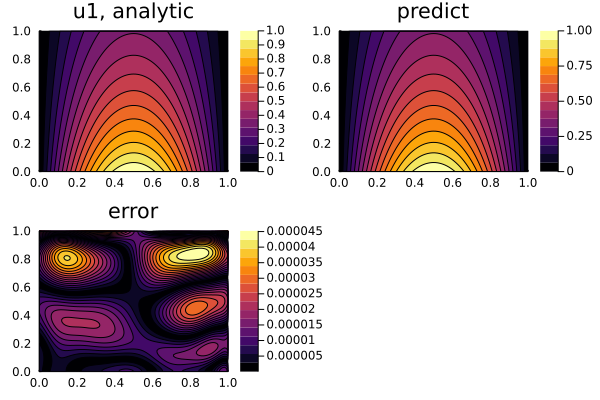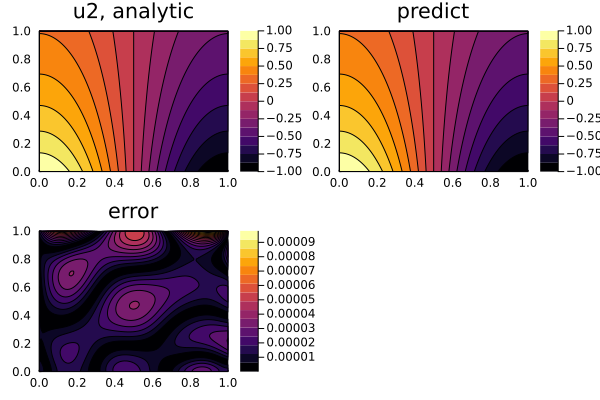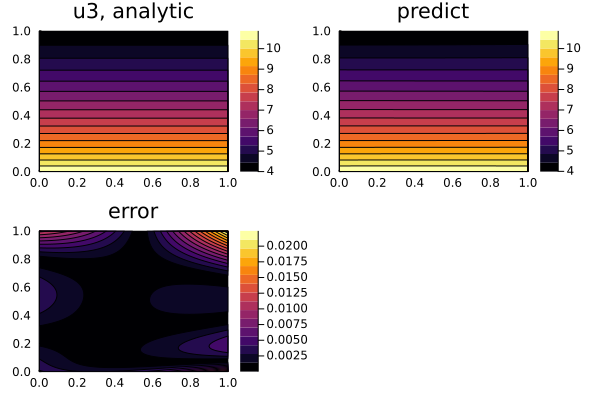## Comparison of the second numerical derivative and numerical + neural network derivative